# How Not to Design a Risk Matrix—Cox’s Axioms

## Introduction

The most widely cited paper on risk matrix design is by Cox (2008). It includes a design methodology based on three axiomatic constraints that Cox suggests risk matrices should satisfy. Cox applies the axioms to produce designs for risk matrices of size 3x3, 4x4, and 5x5. In this article, we review the method and show that it leads to results that are not useful in practice.

## Cox's Axioms

In explaining the design method of Cox, we will adopt “probability” and “impact” as the default names of the two axes of the risk matrix.

Cox assumes that the two axes range from 0 to 1 and are equally subdivided into a number of intervals. For example, for a 4x4 matrix, the axes would be as follows: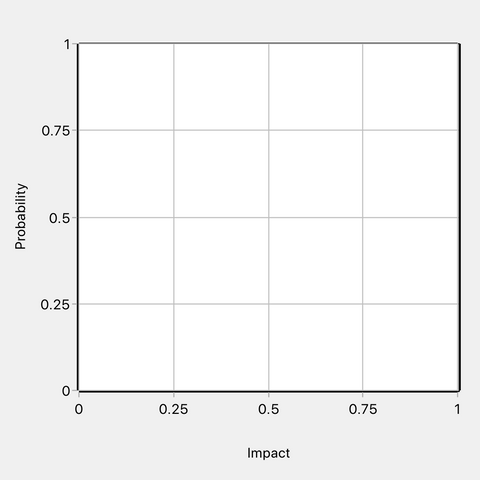Figure 1: 4x4 risk matrix axes

On the probability axis, 0 represents impossibly while 1 represents certainty. On the consequence axis, 0 represents no impact and 1 represents the worst possible adverse impact. The consequence axis tick mark values could be multiplied by any positive scaling factor without changing the mathematical structure.

Cox assumes that risk is calculated as a function of probability and consequence, for example, as the product of the two variables.

Cox proposes that a risk matrix coloring pattern must satisfy three axiomatic constraints. The three constraints he calls Weak Consistency, Betweenness and Consistent Coloring. The following definitions are from Cox’s paper (note he assumes a risk matrix with three colors—red for the top risk category, green for the bottom category, and yellow as an intermediate color):

DEFINITION OF WEAK CONSISTENCY: A risk matrix with more than one “color” (level of risk priority) for its cells satisfies weak consistency with a quantitative risk interpretation if points in its top risk category represent higher quantitative risks than points in its bottom category.

DEFINITION OF BETWEENNESS: A risk matrix satisfies the axiom of betweenness if every positively sloped line segment that lies in a green cell at its lower (left) end and in a red cell at its upper (right) end passes through at least one intermediate cell (meaning one that is neither green nor red) between them.

DEFINITION OF CONSISTENT COLORING: (1) A cell is red if it contains points with quantitative risks at least as high as those in other red cells (and does not contain points with quantitative risk as small as those in any green cell). (2) A cell is colored green if it contains some points with risks at least as small as those in other green cells (and does not contain points with quantitative risks as high as those in any red cell). (3) A cell is colored an intermediate color (neither red nor green) only if either (a) it lies between a red cell and a green cell; or (b) it contains points with quantitative risks higher than those in some red cells and also points with quantitative risks lower than those in some green cells.

According to Cox, the three axioms taken together imply that there is only one possible coloring for a 3x3 or 4x4 matrix, and only two possible colorings for a 5x5 matrix. Let’s take a 4x4 risk matrix as an example. Cox states that the only possible coloring is as shown in Figure 2 below.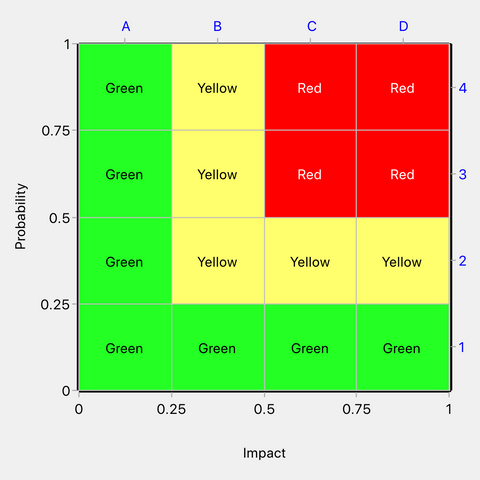Figure 2: The only permissible coloring of a 4x4 risk matrix according to Cox

A good way to understand the three axioms is to consider how the above risk matrix satisfies them.

Weak Consistency. Points in the green cells correspond to quantitative risks from 0 to 0.25. Risks in the red cells correspond to quantitative risks from 0.25 to 1. Points in the top risk category therefore represent risks that are higher than (or equal to) those in the bottom category. Cox’s definition of Weak Consistency (see above) does not mention the possibility that risks in the bottom and top categories could be equal but, from his 4x4 example, it appears that this is acceptable and does not violate Weak Consistency.

Betweenness. It is not possible to draw a line from a green cell to a red cell without passing through at least one yellow cell. Therefore, Betweenness is satisfied.

Consistent Coloring. Inspection shows that the Consistent Coloring axiom is satisfied. But note, the Consistent Coloring axiom contains within it several rules numbered (1), (2), (3)(a) and (3)(b). An interesting feature is that according to Consistent Coloring rule (2), cell B2 should have been green but it has been taken as yellow to satisfy Consistent Coloring rule (3)(a). Rule (3)(a) appears to have been included to allow Betweenness to take precedence over what would otherwise be deemed consistent coloring. In other words, it appears that rules (2) and (3)(a) are in conflict for cell B2, with rule (3)(a) being used as the final determinant of its color.

Note that the Consistent Coloring axiom is very restrictive over when a cell may be colored yellow. It may be yellow according to Rule (3)(a) when it lies between a green cell and a red cell (i.e. when a yellow cell is introduced to satisfy Betweenness). A cell may also be yellow according to Rule (3)(b) when it contains points with quantitative risks higher than those in some red cells and also points with quantitative risks lower than those in some green cells. Due to the restrictive circumstances in which a cell may be colored yellow, many coloring patterns are impermissible, for example, that shown in Figure 3 below.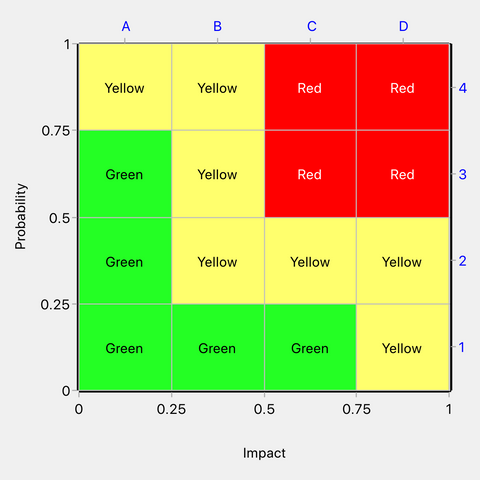Figure 3: An example of a coloring pattern that would be impermissible under the Cox axioms

The above matrix is impermissible under the Cox axioms because yellow cells A4 and D1 comply neither with Consistent Coloring rule (3)(a) nor with (3)(b).

Now we will ask what the one and only permissible design for a 4x4 matrix under the Cox axioms implies about risk priority levels. We have seen that green cells span risks from 0 to 0.25 and red cells span the range 0.25 to 1. Thus, when a risk falls in the red zone, we know it is larger than or equal to 0.25, and when it falls in the green zone it is less than or equal to 0.25. The matrix is therefore useful for discriminating between risks according to whether they are above or below the value of 0.25. We can see that the yellow zone overlaps with both green and red by spanning the risk range from 0.0625 to 0.5. When a risk falls in the yellow zone, we learn nothing about its relationship to a risk threshold of 0.25.

Cox does not explain how the yellow zone should be interpreted. Some points in the yellow cells correspond to risks as high as some points in red cells, while other points in yellow cells correspond to risks as low as some points in green cells. Yellow could be assumed to be an intermediate risk level between red and green but we can see that it overlaps with both red and green in terms of risk range, so it is not actually an intermediate level.

The Cox 4x4 risk matrix design is more or less compatible with the quantitative model shown in Figure 4 that classifies risks as red or green according to their relationship to an iso-risk contour of value 0.25. Simply color the cells that contain both red and green coloring as yellow and you will have the Cox design—except that Cox forces cell B2 to be yellow rather than green on account of Betweenness and the Consistent Coloring Rule (3)(a). Without forcing the color of cell B2, we would have the matrix in Figure 5, which is the same as Cox’s design except for cell B2.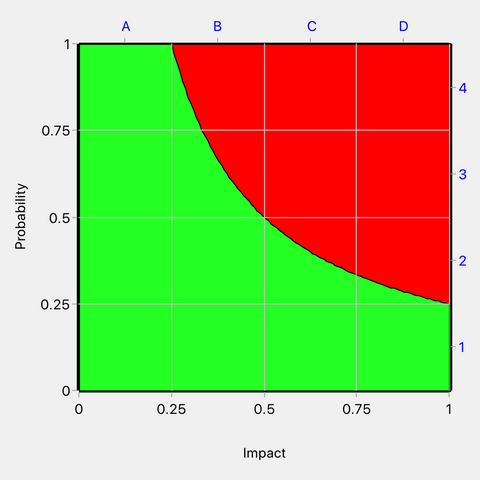Figure 4: A quantitative model compatible with the Cox 4x4 risk matrix design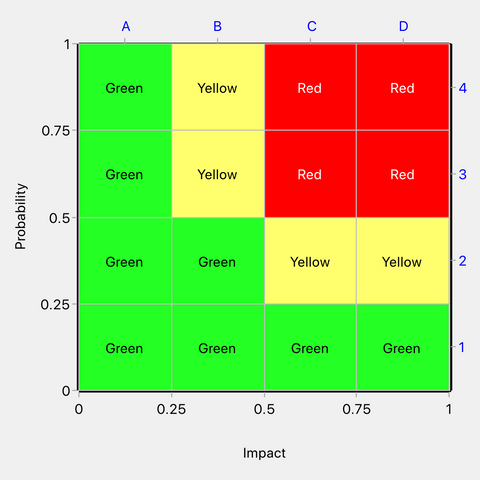Figure 5: Matrix compatible with the quantitative model in Figure 4

It seems that the only valid use of the Cox 4x4 matrix is to tell us whether a risk is above or below a threshold of 0.25. It will do this accurately when the risk falls in the red or green zone but provides no useful information when the risk falls in the yellow zone. Therefore, a logical development of the design is to approximate the yellow cells as either green or red. Let us approximate the yellow cells as red. Our design now evolves to: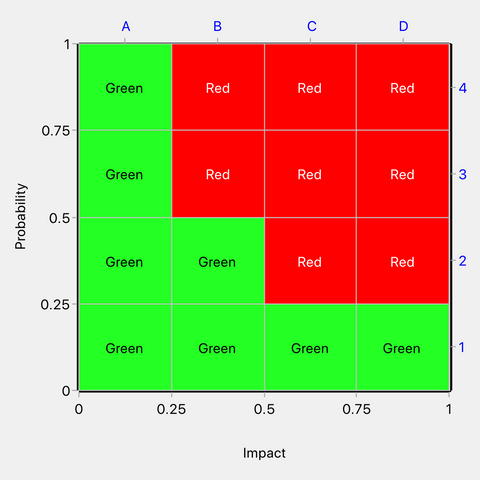Figure 6: Yellow cells approximated as red

Suppose that risk points are uniformly distributed in Figure 6. We can simulate a large number of such points and determine how many are correctly ranked according to the underlying model in Figure 4. We find that 90% of points will be correctly ranked and 10% will have their rank overestimated, which is a respectable performance for an approximate tool.

But the single risk level of 0.25 implied in the Cox 4x4 matrix is unlikely to be of any use to the decisionmaker. Risks in the domain of the matrix can range from 0 at the bottom left to 1 at top right. The decisionmaker might be interested in a value for the risk threshold anywhere in this range. Also, in practice, the decisionmaker will likely want to use more than one risk threshold, e.g. to divide risks into three or more categories. Cox’s 4x4 matrix design relates to a single risk threshold fixed at 0.25 by the application of the three axiomatic constraints.

## Conclusions

The three Cox axioms overly constrain risk matrix design resulting in a single implied risk threshold between the top and bottom risk categories. We demonstrated this to be the case for the 4x4 matrix, but it is also the case for the 3x3 and 5x5 risk matrix designs in the Cox paper.

The Cox axioms cannot be used to develop a design that is compatible with arbitrary risk threshold values specified by the decisionmaker.

The Cox axioms are not useful for practical risk matrix design.

## References

Cox, L. A., Jr. (2008). What's wrong with risk matrices? Risk Analysis, 28(2), 497-512. doi:10.1111/j.1539-6924.2008.01030.x